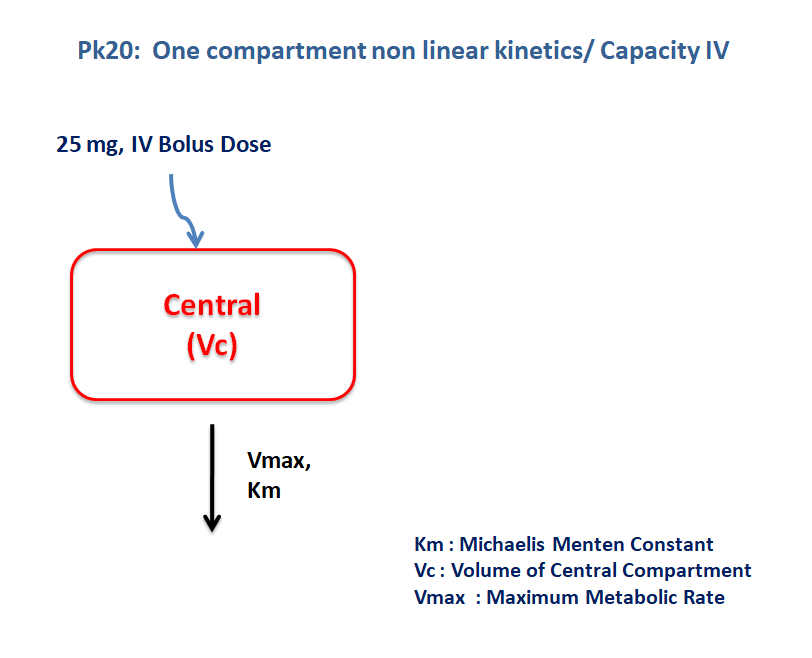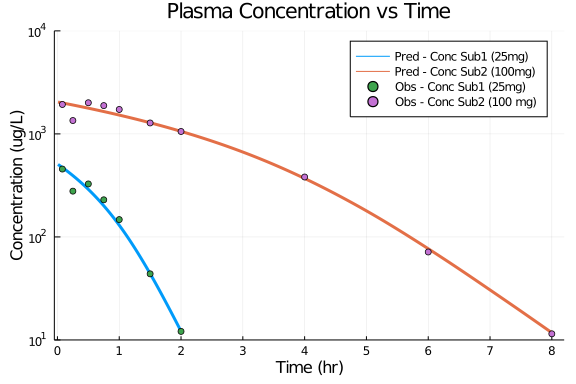# Exercise PK20 - Capacity IV - Noninear Kinetics

### Background

• Structural Model - One Compartment Model with Nonlinear elimination.

• Route of administration - IV bolus

• Dosage Regimen - 25 mg, 100 mg

• Number of Subjects - 2### Learing Outcome

This is a one compartment model for IV bolus dose with capacity limited elimination. Concentration time profile was obtained for two subjects with different dosage regimen and different Vmax and Km values.

### Objectives

In this tutorial, you will learn how to build one compartment model for IV bolus dose with capacity limited elimination.

### Libraries

call the "necessary" libraries to get start.

using Pumas
using Plots
using CSV
using StatsPlots
using Random


### Model

pk_20        = @model begin
@param   begin
tvvc     ∈ RealDomain(lower=0)
tvkm     ∈ RealDomain(lower=0)
tvvmax   ∈ RealDomain(lower=0)
Ω        ∈ PDiagDomain(3)
σ²_prop  ∈ RealDomain(lower=0)
end

@random begin
η        ~ MvNormal(Ω)
end

@pre begin
Vc       = tvvc * exp(η)
Km       = tvkm * exp(η)
Vmax     = tvvmax * exp(η)
end

@dynamics begin
Central' = - (Vmax * (Central/Vc)/(Km + (Central/Vc)))
end

@derived begin
cp       = @. Central/Vc
dv       ~ @. Normal(cp, sqrt(cp^2*σ²_prop))
end
end

PumasModel
Parameters: tvvc, tvkm, tvvmax, Ω, σ²_prop
Random effects: η
Covariates:
Dynamical variables: Central
Derived: cp, dv
Observed: cp, dv


### Parameters

The parameters are as given below. tv represents the typical value for parameters.

• Km - Michaelis menten Constant (μg/L)

• Vc - Volume of Central Compartment (L),

• Vmax - Maximum rate of metabolism (μg/hr),

• Ω - Between Subject Variability,

• σ - Residual error

Note:-

• param1 are the parameter values for Subject 1

• param2 are the parameter values for Subject 2

param1 = (tvkm    = 261.736,
tvvmax  = 36175.1,
tvvc    = 48.9892,
Ω       = Diagonal([0.0,0.0,0.0]),
σ²_prop = 0.01)

(tvkm = 261.736, tvvmax = 36175.1, tvvc = 48.9892, Ω = [0.0 0.0 0.0; 0.0 0.
0 0.0; 0.0 0.0 0.0], σ²_prop = 0.01)

param2 = (tvkm    = 751.33,
tvvmax  = 36175.1,
tvvc    = 48.9892,
Ω       = Diagonal([0.0,0.0,0.0]),
σ²_prop = 0.01)

(tvkm = 751.33, tvvmax = 36175.1, tvvc = 48.9892, Ω = [0.0 0.0 0.0; 0.0 0.0
0.0; 0.0 0.0 0.0], σ²_prop = 0.01)


### Dosage Regimen

• Subject1 - receives an IV dose of 25 mg or 25000 μg

• Subject2 - recieves an IV dose of 100 mg or 100000 μg

ev1  = DosageRegimen(25000, time=0, cmt=1)
sub1 = Subject(id=1, events=ev1)
ev2  = DosageRegimen(100000, time=0, cmt=1)
sub2 = Subject(id=2, events=ev2)


### Simulation

Simulate the plasma drug concentration for both the subjects

## Subject 1
Random.seed!(123)
sim_sub1 = simobs(pk_20, sub1, param1, obstimes=0.01:0.01:2)
df1      = DataFrame(sim_sub1)

##Subject 2
Random.seed!(123)
sim_sub2 = simobs(pk_20, sub2, param2, obstimes=0.01:0.01:8)
df2      = DataFrame(sim_sub2)


## Plot

df1_dv = filter(x -> x.time in [0.08,0.25,0.5,0.75,1,1.5,2], df1)
df2_dv = filter(x -> x.time in [0.08,0.25,0.5,0.75,1,1.5,2,4,6,8], df2)

@df df1 plot(:time, :cp, yaxis=:log,
title= "Plasma Concentration vs Time", label = "Pred - Conc Sub1 (25mg)",
xlabel= "Time (hr)", ylabel = "Concentration (ug/L)", linewidth=3,
xticks=[0,1,2,3,4,5,6,7,8], xlims=(-0.05,8.2), yticks=[10,100,1000,10000], ylims=(10,10000))
@df df2 plot!(:time, :cp, label = "Pred - Conc Sub2 (100mg)", linewidth=3)
@df df1_dv scatter!(:time, :dv, label = "Obs - Conc Sub1 (25mg)")
@df df2_dv scatter!(:time, :dv, label = "Obs - Conc Sub2 (100 mg)")